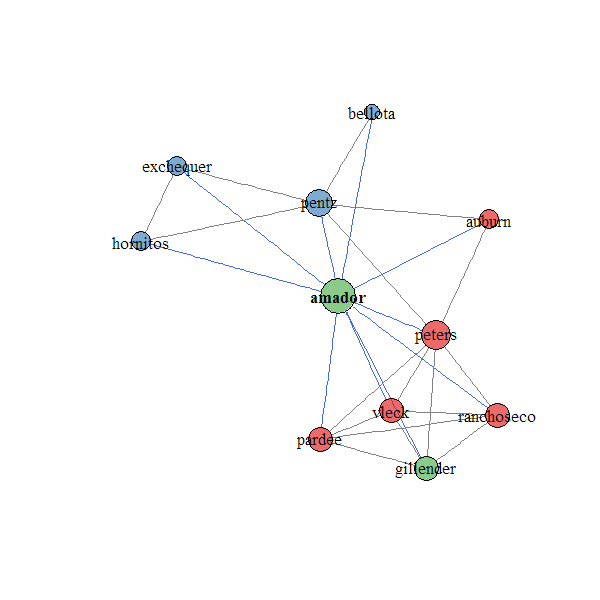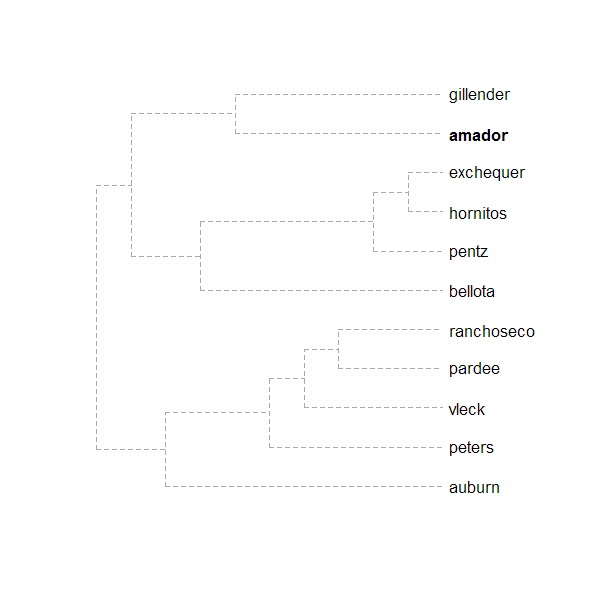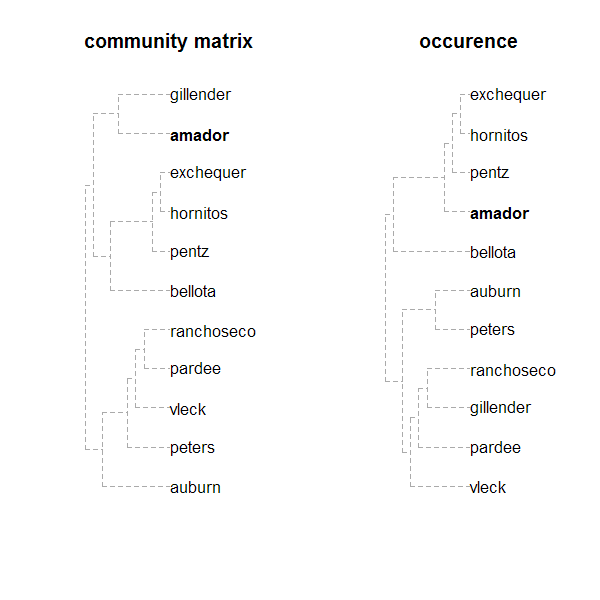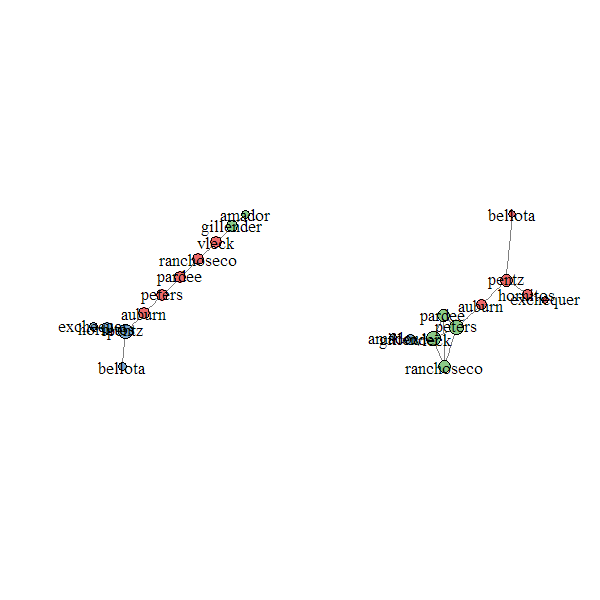plotSoilRelationGraph {sharpshootR} R Documentation

## Plot a component relation graph

### Description

Plot a component relation graph based on an adjacency or similarity matrix.

### Usage

```plotSoilRelationGraph(m, s='', plot.style='network', spanning.tree=NULL,
del.edges=NULL, vertex.scaling.factor=2, edge.scaling.factor=1,
edge.transparency=1, edge.col=grey(0.5), edge.highlight.col='royalblue',
g.layout=layout.fruchterman.reingold,
...)
```

### Arguments

 `m` adjacency matrix `s` central component; an empty character string is interpreted as no central component `plot.style` plot style ('network', or 'dendrogram'), or 'none' for no graphical output `spanning.tree` plot the minimim or maximum spaning tree ('min', 'max'), or, max spanning tree plus edges with weight greater than the n-th quantile specified in 'spanning.tree'. See details and examples. `del.edges` optionally delete edges with weights less than the specified quantile (0-1) `vertex.scaling.factor` scaling factor applied to vertex size `edge.scaling.factor` optional scaling factor applied to edge width `edge.transparency` optional transparency setting for edges (0-1) `edge.col` edge color, applied to all edges `edge.highlight.col` edge color applied to all edges connecting to component named in `s` `g.layout` an igraph layout function, defaults to `layout.fruchterman.reingold` `...` further arguments passed to plotting function

### Details

Vertex size is based on a normalized index of connectivity: size = sqrt(degree(g)/max(degree(g))) * scaling.factor. Edge width can be optionally scaled by edge weight by specifying an `edge.scaling.factor` value. The maximum spanning tree represents a sub-graph where the sum of edge weights are maximized. The mimimum spanning tree represents a sub-graph where the sum of edge weights are minimized. The maximum spanning tree is likely a more useful simplification of the full graph, in which only the strongest relationships (e.g. most common co-occurrences) are preserved.

The maximum spanning tree + edges with weights > n-th quantile is an experimental hybrid. The 'backbone' of the graph is created by the maximum spanning tree, and augmented by 'strong' auxillary edges– defined by a value between 0 and 1.

### Value

an igraph 'graph' object is invisibly returned

### Note

This function is a work in progress, ideas welcome.

D.E. Beaudette

### Examples

``````# load sample data set

# create weighted adjacency matrix (see ?component.adj.matrix for details)

# plot network diagram, with Amador soil highlighted
````````````# dendrogram representation
````````````# compare methods

par(mfcol=c(1,2))
title('community matrix')
title('occurence')
````````````# investigate max spanning tree
plotSoilRelationGraph(m, spanning.tree='max')

# investigate max spanning tree + edges with weights > 75-th pctile
plotSoilRelationGraph(m, spanning.tree=0.75)
````````````## Not run:
##D # get similar data from soilweb, for the Pardee series
##D s <- 'pardee'
##D u <- url(URLencode(paste(
##D 'http://casoilresource.lawr.ucdavis.edu/soil_web/reflector_api/soils.php?',
##D 'what=soil_series_component_query&q_string=', s, sep='')))
##D
##D # fetch data
##D d <- read.table(u, sep='|', header=TRUE, stringsAsFactors=FALSE)
##D
##D # normalize component names
##D d\$compname <- tolower(d\$compname)
##D
##D # keep only major components
##D d <- subset(d, subset=compkind == 'Series')
##D
##D # build adj. matrix and plot
##D m <- component.adj.matrix(d)
##D plotSoilRelationGraph(m, s=s, plot.style='dendrogram')
##D
##D # alter plotting style, see ?plot.phylo
##D plotSoilRelationGraph(m, s=s, plot.style='dendrogram', type='fan')
##D plotSoilRelationGraph(m, s=s, plot.style='dendrogram', type='unrooted', use.edge.length=FALSE)
## End(Not run)
``````

[Package sharpshootR version 0.8-4 Index]